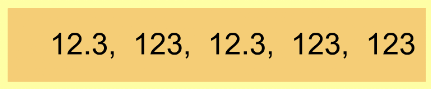Finding the Mode 1
Set 1 (10 Questions)
Students organize, represent, and interpret numerical and categorical data and clearly communicate their findings. Know the concepts of mean, median, and mode; compute and compare simple examples to show that they may differ.
From Mr. Anker Tests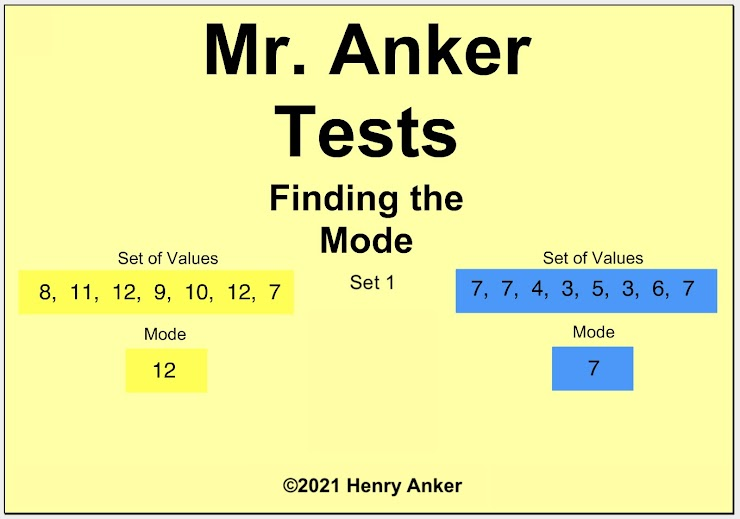Finding the Mode Help Slide 1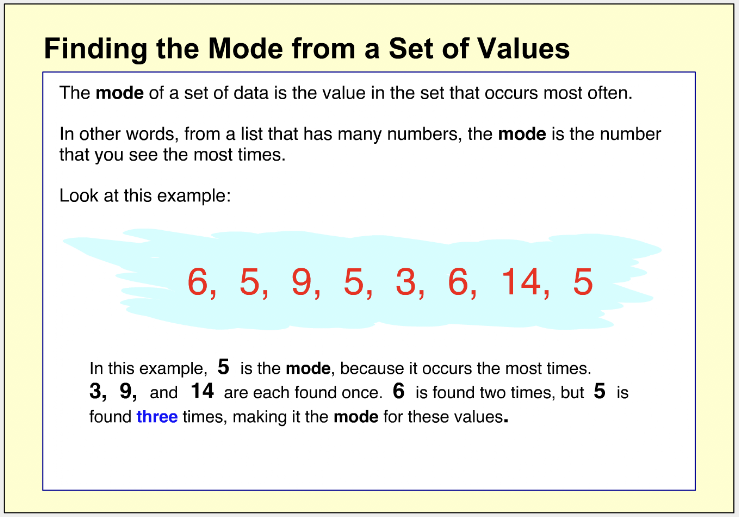Finding the Mode Help Slide 2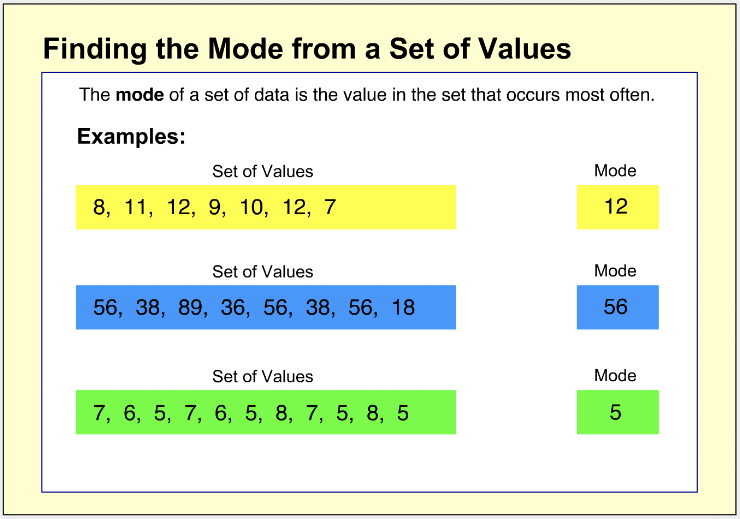Finding the Mode Help Slide 3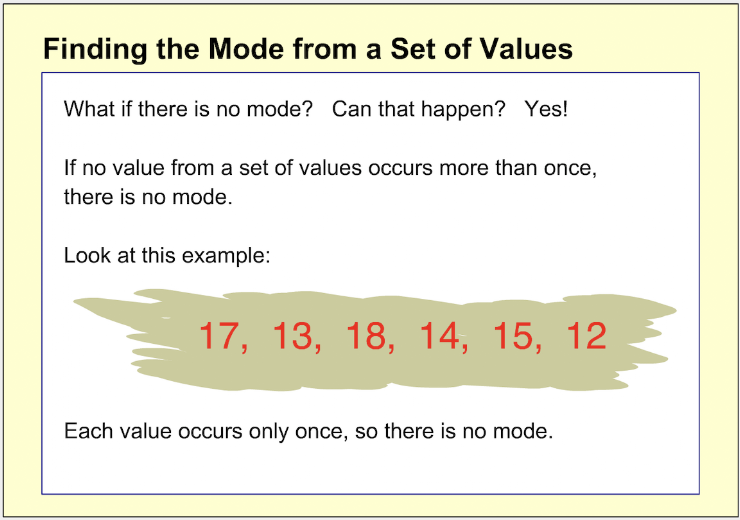Finding the Mode Help Slide 4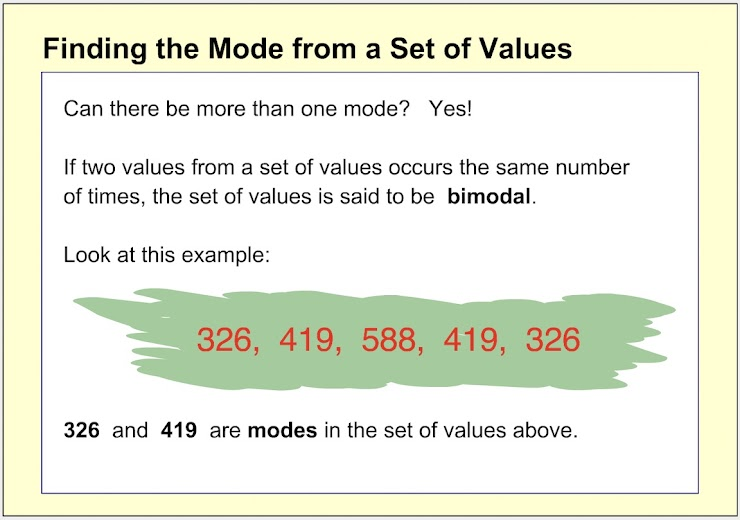Finding the Mode Help Slide 5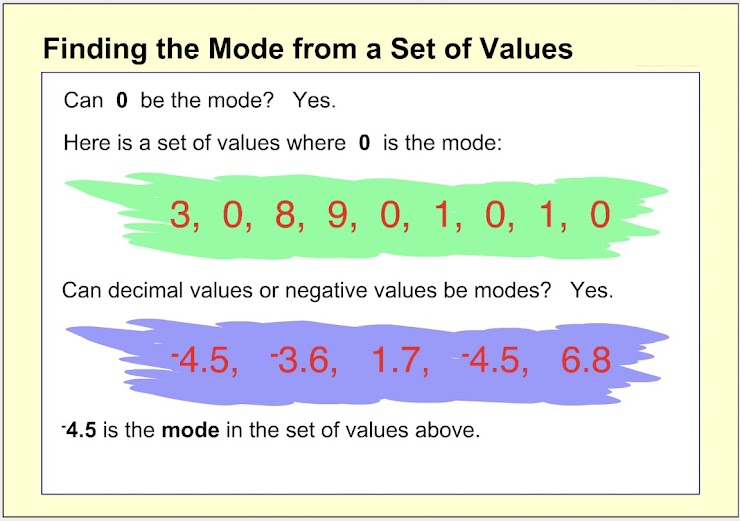1. Which choice below is the mode for the set of values shown? *
1 point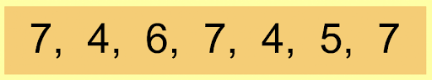Remember, the mode is the value that occurs the most times.
2. Which choice below is the mode for the set of values shown? *
1 point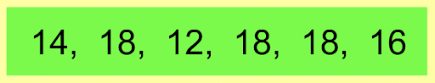3. Which choice below is the mode for the set of values shown? *
1 point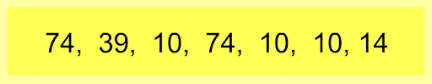4. Which choice below is the mode for the set of values shown? *
1 point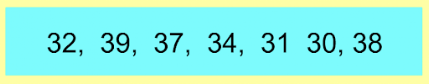5. On the line below, enter the mode for the set of values shown. *
1 point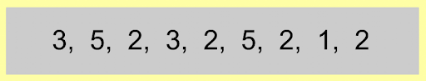6. On the line below, enter the mode for the set of values shown. *
1 point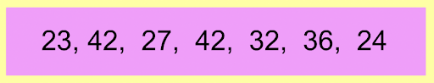7. On the line below, enter the mode for the set of values shown. *
1 point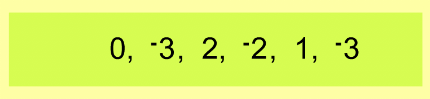8. On the line below, enter the mode for the set of values shown. *
1 point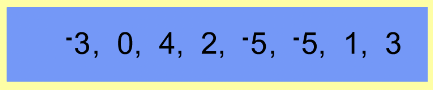9. On the line below, enter the mode for the set of values shown. *
1 point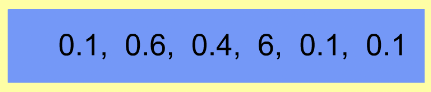10. On the line below, enter the mode for the set of values shown. *
1 point